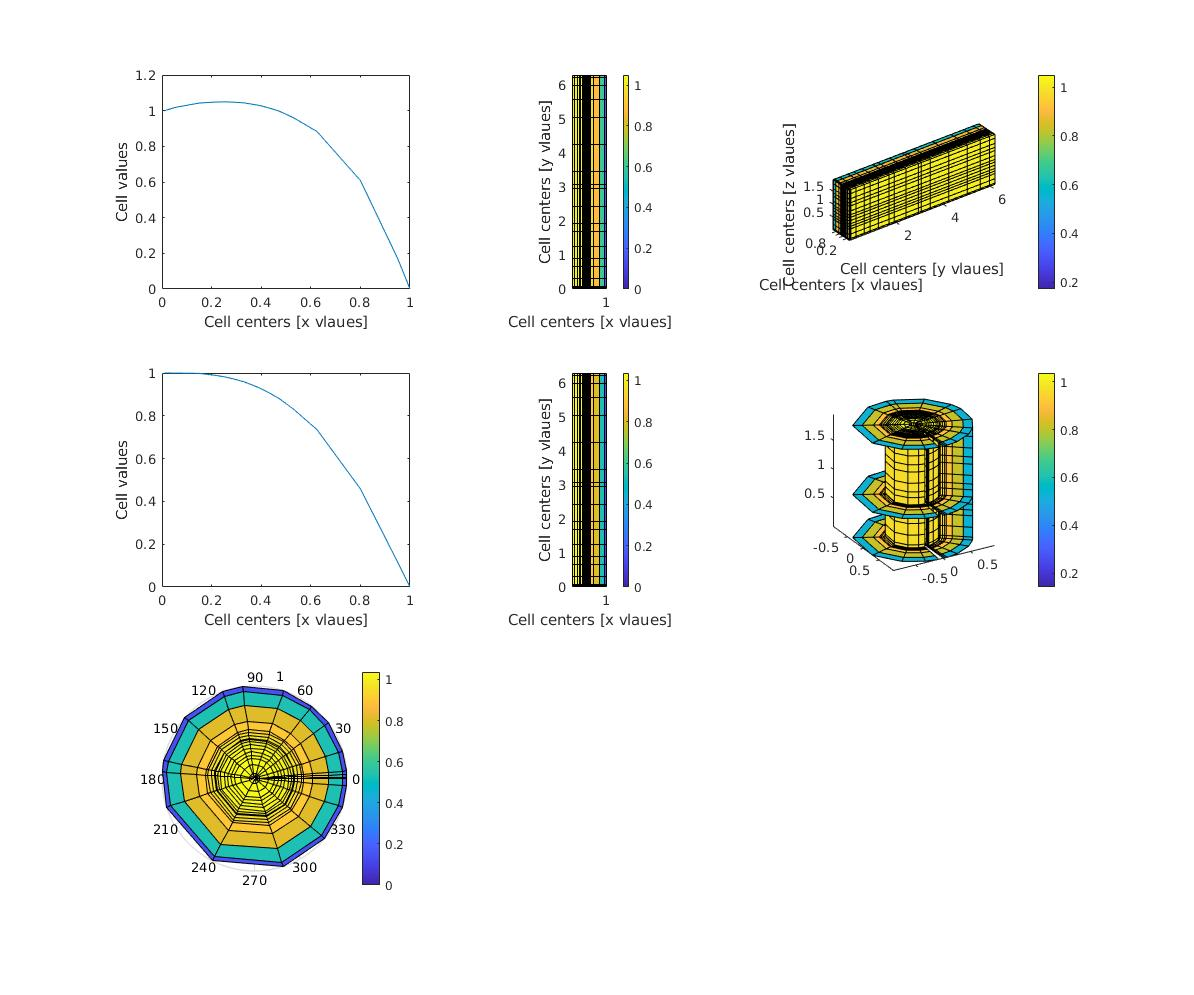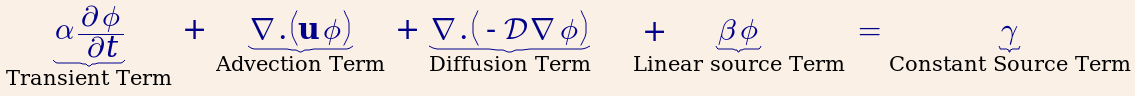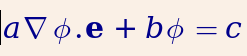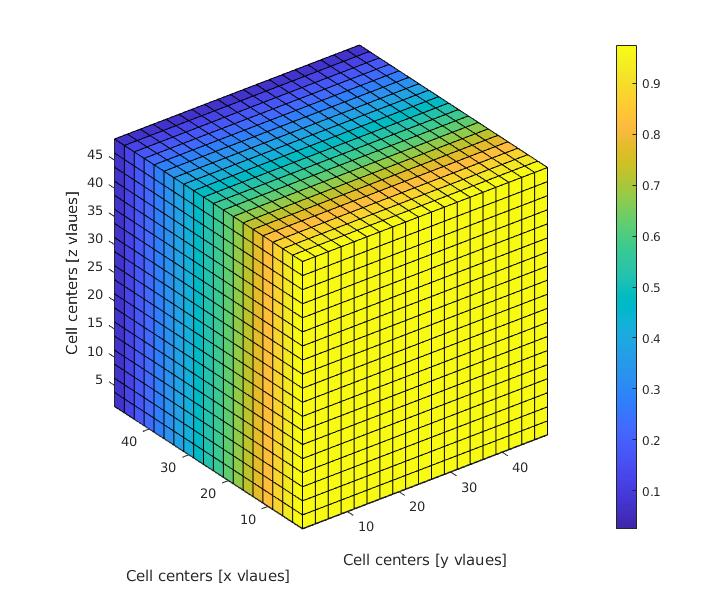## A Simple Finite Volume Solver for Matlab

Version 2.1.1.0 (1010 KB) by
A simple yet general purpose FVM solver for transient convection diffusion PDE

Updated 28 Nov 2022

From GitHub

# FVTool: Finite volume toolbox for Matlab

This is a finite volume (toy) toolbox for chemical/petroleum engineers. Right now, it can solve a transient convection-diffusion equation with variable velocity field/diffusion coefficients. The discretization schemes include:

• central difference diffusion term
• central difference convection term
• upwind convection term
• TVD convection term with various flux limiters
• transient term
• Dirichlet, Neumann, Robin, and periodic boundary conditions## Which equation do you solve?

You can solve the following PDE (or a subset of it):with the following boundary conditions:Believe it or not, the above equations describe the majority of the transport phenomena in chemical and petroleum engineering and similar fields.

## How to start

Download the package, start matlab, and run FVToolStartUp

## Inspiration

I started writing this tool after playing with FiPy, an amazing python-based finite volume solver. This matlab solver is not a clone, and indeed very limited compared to FiPy. I wrote it to have a very handy tool for testing new ideas (new mathematical models) by solving them in 1D uniform Cartesian grids. Then I extended the code to

• 2D Cartesian
• 3D Cartesian
• 2D axisymmetric (cylindrical, r, z)
• 3D cylindrical (r, theta, z)

I have overloaded some of the matlab operators to simplify the switch from 1D codes to 2D and 3D.

### A simple example

You can solve a diffusion equation, i.e., $\nabla. (-D \nabla \phi) = 0$ by running the following code in Matlab:

clc
L = 50;  % domain length
Nx = 20; % number of cells
m = createMesh1D(Nx, L);
BC = createBC(m); % all Neumann boundary condition structure
BC.left.a(:) = 0; BC.left.b(:)=1; BC.left.c(:)=1; % Dirichlet for the left boundary
BC.right.a(:) = 0; BC.right.b(:)=1; BC.right.c(:)=0; % right boundary
D_val = 1; % value of the diffusion coefficient
D = createCellVariable(m, D_val); % assign the diffusion coefficient to the cells
D_face = harmonicMean(D); % calculate harmonic average of the diffusion coef on the cell faces
Mdiff = diffusionTerm(D_face); % matrix of coefficients for the diffusion term
[Mbc, RHSbc] = boundaryCondition(BC); % matrix of coefficients and RHS vector for the BC
M = Mdiff + Mbc; % matrix of coefficients for the PDE
c = solvePDE(m,M, RHSbc); % send M and RHS to the solver
visualizeCells(c); % visualize the results

change the third line to m = createMesh2D(Nx,Nx, L,L); or m = createMesh3D(Nx,Nx,Nx, L,L,L); and see the outcome for yourself.## Examples

There are a few simple examples in the Tutorial folder. You can also find a few more advanced examples (water injection into a heterogeneous oil field, two nonlinear PDEs, coupled fully implicit solution) in the Advanced folder.

## Documents

Find some preliminary documents here.

## But Matlab is not a free software?

You can use the code in octave. The new (object oriented) version of the code works in Octave 4.0 (with the new classdef function).
I've re-written the code in Julia. It works fine, but the visualization on Windows OS has still some issues.

## Questions and bug reports

You can ask your questions by creating a new issue here, or by writing a comment in my blog. You can also ask your question in the Matlab file exchange page of this code. I truly appreciate your feedback and/or contribution.

## How to cite:

If you have used the package in your work and you find it usefull, please cite it as:

@misc{ali_akbar_eftekhari_2015_32745,
author       = {Ali Akbar Eftekhari and Kai Schüller and Ferran Brosa Planella and Martinus Werts and Behzad Hosseinzadeh},
title        = {FVTool: a finite volume toolbox for Matlab},
month        = oct,
year         = 2015,
doi          = {10.5281/zenodo.32745},
url          = {https://doi.org/10.5281/zenodo.32745}
}


I will also appreciate it if you write me a couple of lines about how you have used it in your research. It encourages me to maintain the code.

### Cite As

Eftekhari, A.A. et al. (2015). FVTool: a finite volume toolbox for Matlab. Zenodo. http://doi.org/10.5281/zenodo.32745

##### MATLAB Release Compatibility
Created with R2014a
Compatible with any release
##### Platform Compatibility
Windows macOS Linux
##### Acknowledgements

Inspired by: IAPWS_IF97

### Community Treasure Hunt

Find the treasures in MATLAB Central and discover how the community can help you!

Start Hunting!

#### Examples/Validation

Version Published Release Notes
2.1.1.0

2.1.0.0

Citation information and some clean up

2.0.0.0

showdemo function is not available. I will update it later.

1.4.0.0

added support for 2D radial (r, theta) and 3D cylindrical (r, thetta, z)

1.3.0.0

updated descriptions

1.2.0.0